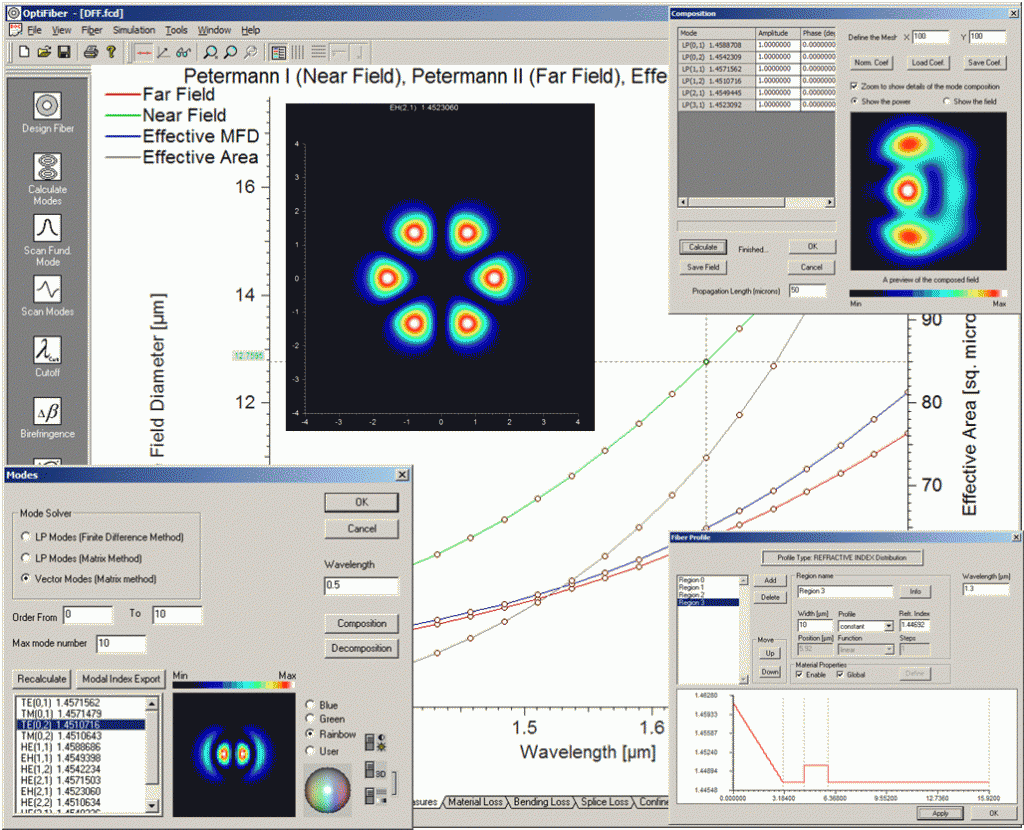Meshless Mode Solversfor LP and Vector Modes: Optical fibers may consist of an arbitrary number of concentric layers of lossless materials, and graded index fibers can be approximated using a sequence of constant index layers. OptiFiber 2.0 mode solvers find an exact solution based on matching boundary conditions at layer boundaries instead of relying on meshes to approximate the structure.

These advanced mode solvers should be especially useful for multimode fiber calculations, where there are many modes in the spectrum.

Another advantage of the meshless mode solver is the calculation of fields far from the fiber. Meshing introduces finite difference errors of a certain level, and fields weaker than the differencing error cannot be calculated. The meshless mode solvers, on the other hand, have the correct asymptotic behavior far from the fiber, and can calculate fields of magnitude 10-15 or less.

This feature is in addition to the existing mode solvers in OptiFiber.

Propagation over Distance: OptiFiber allows users to decompose an arbitrary field into the modes of a multimode fiber. It calculates the complex coefficients of the modes for the arbitrary field. Similarly, given the amplitude of a set of modes, OptiFiber can display the sum (composition of modes).

OptiFiber 2.0 can also calculate this multimode field after propagating down the fiber by a specified distance. The user enters a distance. Each of the complex coefficients of the modes is multiplied by the appropriate phase factor, and the new field pattern is displayed in the preview box.

KEY APPLICATIONS

• Assess parameters, sensitivities, and tolerances
• Fiber mode solving of LP or Vector modes by Finite Difference or by Transfer Matrix Methods
• Analysis of measured fiber profiles from instruments such as the EXFO NR-9200
• Single mode fiber designs such as Corning SMF-28, dispersion flattened or shifted fibers.
• Multimode fiber design, such as 50/125 m and 62.5/125 m silica fibers.
• Visualization of multimode interference patterns with propagation
• Automatic parameter scanning
• Fiber Sensor design
• Calculation of birefringence and PMD from intrinsic or extrinsic perturbations.